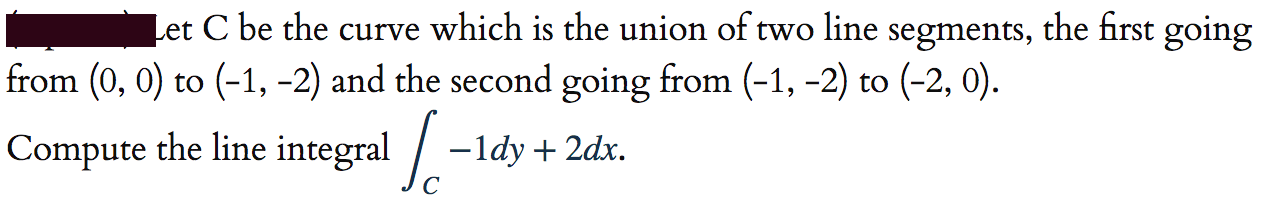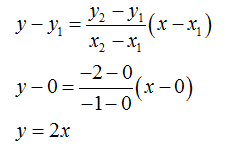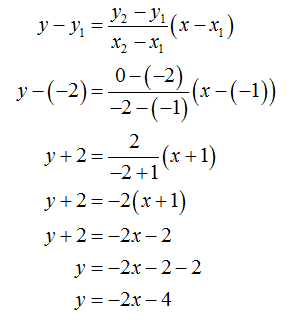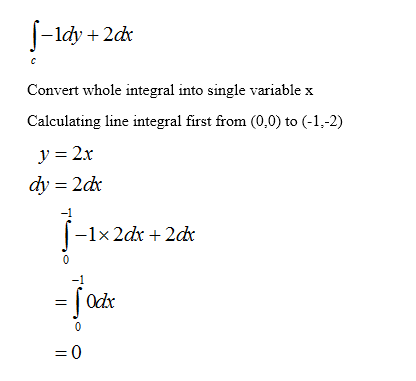# Let C be the curve which is the union of two line segments, the first goingfrom (0, 0) to (-1, -2) and the second going from (-1, -2) to (-2, 0).Compute the line integral / --ldy + 2dx.

Question
75 viewshelp_outlineImage TranscriptioncloseLet C be the curve which is the union of two line segments, the first going from (0, 0) to (-1, -2) and the second going from (-1, -2) to (-2, 0). Compute the line integral / - -ldy + 2dx. fullscreen
check_circle

star
star
star
star
star
1 Rating
Step 1

Given:

The curve C is the union of two-line segments, first going from (0,0) to (-1,-2) and the second going from (-1,-2) to (-2,0)

Equation of line passing through from (0,0) to (-1,-2) is given byStep 2

Equation of line passing through from (-1,-2) to (-2,0) is given byStep 3

The line integral...

### Want to see the full answer?

See Solution

#### Want to see this answer and more?

Solutions are written by subject experts who are available 24/7. Questions are typically answered within 1 hour.*

See Solution
*Response times may vary by subject and question.
Tagged in

### Calculus